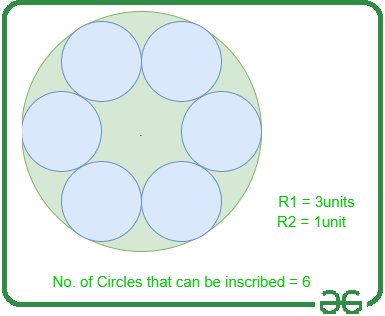Related Articles

# Number of smaller circles that can be inscribed in a larger circle

• Last Updated : 22 Apr, 2021

Given two positive integers R1 and R2, where R1 and R2 represent the radius of the larger and smaller circles respectively, the task is to find out the number of smaller circles that can be placed inside the larger circle such that the smaller circle touches the boundary of the larger circle.

Examples:

Input: R1 = 3, R2 = 1
Output: 6
Explanation: The radii of the circles are 3 and 1. Therefore, the circle of radius 1 can be inscribed in the circle of radius 3.From the above representation, the total number of smaller circles that can be inscribed with touching of the boundary of the larger circle is 6.

Input: R1 = 5, R2 = 4
Output: 1

Approach: The given problem can be solved by finding the angle that the smaller circle of radius R2 makes at the centre of the circle with radius R1 and then divide it by 360 degrees
Follow the steps below to solve the given problem:

• If the value of R1 is less than R2, then it is impossible to inscribe a single circle. Therefore, print 0.
• If the value of R1 is less than 2 * R2, i.e. if the diameter of the smaller circle is greater than the radius of the larger circle, then only one circle can be inscribed. Therefore, print 1.
• Otherwise, find the angle made by the smaller circle at the centreof the larger circle using the below formula and then, divide by 360 degrees to get the total number of circles that can be inscribed and print that value.Below is the implementation of the above approach:

## C++

 // C++ program for the above approach #include using namespace std; // Function to count number of smaller// circles that can be inscribed in// the larger circle touching its boundaryint countInscribed(int R1, int R2){    // If R2 is greater than R1    if (R2 > R1)        return 0;     // Stores the angle made    // by the smaller circle    double angle;     // Stores the ratio    // of R2 / (R1 - R2)    double ratio;     // Stores the count of smaller    // circles that can be inscribed    int number_of_circles = 0;     // Stores the ratio    ratio = R2 / (double)(R1 - R2);     // If the diameter of smaller    // circle is greater than the    // radius of the larger circle    if (R1 < 2 * R2) {        number_of_circles = 1;    }     // Otherwise    else {         // Find the angle using formula        angle = abs(asin(ratio) * 180)                / 3.14159265;         // Divide 360 with angle        // and take the floor value        number_of_circles = 360                            / (2                               * floor(angle));    }     // Return the final result    return number_of_circles;} // Driver Codeint main(){    int R1 = 3;    int R2 = 1;     cout << countInscribed(R1, R2);     return 0;}

## Java

 // Java program for the above approachimport java.util.*; class GFG{  // Function to count number of smaller// circles that can be inscribed in// the larger circle touching its boundarystatic int countInscribed(int R1, int R2){         // If R2 is greater than R1    if (R2 > R1)        return 0;     // Stores the angle made    // by the smaller circle    double angle;     // Stores the ratio    // of R2 / (R1 - R2)    double ratio;     // Stores the count of smaller    // circles that can be inscribed    int number_of_circles = 0;     // Stores the ratio    ratio = R2 / (double)(R1 - R2);     // If the diameter of smaller    // circle is greater than the    // radius of the larger circle    if (R1 < 2 * R2)    {        number_of_circles = 1;    }     // Otherwise    else    {                 // Find the angle using formula        angle = Math.abs(Math.asin(ratio) * 180) /                3.14159265;         // Divide 360 with angle        // and take the floor value        number_of_circles = (int)(360 /        (2 * Math.floor(angle)));    }         // Return the final result    return number_of_circles;} // Driver Codepublic static void main(String args[]){    int R1 = 3;    int R2 = 1;         System.out.println(countInscribed(R1, R2));}} // This code is contributed by ipg2016107

## Python3

 # Python3 program for the above approachimport math # Function to count number of smaller# circles that can be inscribed in# the larger circle touching its boundarydef countInscribed(R1, R2):         # If R2 is greater than R1    if (R2 > R1):        return 0     # Stores the angle made    # by the smaller circle    angle = 0     # Stores the ratio    # of R2 / (R1 - R2)    ratio = 0     # Stores the count of smaller    # circles that can be inscribed    number_of_circles = 0     # Stores the ratio    ratio = R2 / (R1 - R2)     # If the diameter of smaller    # circle is greater than the    # radius of the larger circle    if (R1 < 2 * R2):        number_of_circles = 1         # Otherwise    else:         # Find the angle using formula        angle = (abs(math.asin(ratio) * 180) /                 3.14159265)                # Divide 360 with angle        # and take the floor value        number_of_circles = (360 / (2 *               math.floor(angle)))         # Return the final result    return number_of_circles # Driver Codeif __name__ == "__main__":     R1 = 3    R2 = 1     print (int(countInscribed(R1, R2))) # This code is contributed by ukasp

## C#

 // C# program for the above approachusing System; class GFG{  // Function to count number of smaller// circles that can be inscribed in// the larger circle touching its boundarystatic int countInscribed(int R1, int R2){         // If R2 is greater than R1    if (R2 > R1)        return 0;     // Stores the angle made    // by the smaller circle    double angle;     // Stores the ratio    // of R2 / (R1 - R2)    double ratio;     // Stores the count of smaller    // circles that can be inscribed    int number_of_circles = 0;     // Stores the ratio    ratio = R2 / (double)(R1 - R2);     // If the diameter of smaller    // circle is greater than the    // radius of the larger circle    if (R1 < 2 * R2)    {        number_of_circles = 1;    }     // Otherwise    else    {                 // Find the angle using formula        angle = Math.Abs(Math.Asin(ratio) * 180) /                3.14159265;         // Divide 360 with angle        // and take the floor value        number_of_circles = (int)(360 /        (2 * Math.Floor(angle)));    }         // Return the final result    return number_of_circles;} // Driver Codepublic static void Main(){    int R1 = 3;    int R2 = 1;         Console.WriteLine(countInscribed(R1, R2));}} // This code is contributed by mohit kumar 29

## Javascript

 
Output:
6

Time Complexity: O(1)
Auxiliary Space: O(1)

Attention reader! Don’t stop learning now. Get hold of all the important mathematical concepts for competitive programming with the Essential Maths for CP Course at a student-friendly price. To complete your preparation from learning a language to DS Algo and many more,  please refer Complete Interview Preparation Course.

My Personal Notes arrow_drop_up